## A microwave oven operates at a frequency of 2400 MHz. The height of the oven cavity is 25 cm and the base measures 30 cm by 30 cm. Assume th

Question

A microwave oven operates at a frequency of 2400 MHz. The height of the oven cavity is 25 cm and the base measures 30 cm by 30 cm. Assume that microwave energy is generated uniformly on the upper surface of the cavity and propagates directly
downward toward the base. The base is lined with a material that completely absorbs microwave energy. The total microwave energy content of the cavity is 0.50 mJ.

A)The power output of the microwave oven is?
B) The intensity of the microwave beam is?
C) The electric field amplitude is?
D) The force on the base due to the radiation is?

in progress 0
6 months 2021-08-10T01:17:57+00:00 1 Answers 1 views 0

power = 600000 W

intensity = 6666666.66 W/m²

Em = 70880.18 N/m

F = 2 ×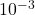N

Explanation:

given data

frequency f = 2400 MHz

height oven cavity h = 25 cm = 0.25 m

base area measures A =  30 cm by 30 cm

total microwave energy content of cavity E = 0.50 mJ = 0.50 ×solution

first, we get here total time taken from top to bottom that is express as

Δt =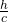……………1

Δt =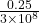Δt = 8.33 ×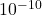s

and

power output will be

power =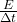…………..2

power =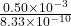power = 600000 W

and

intensity of the microwave beam is

intensity = power output ÷ base area    …………..2

intensity =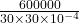intensity = 6666666.66 W/m²

and

electric field amplitude is

as we know intensity  I =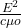……………3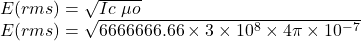E(rms) = 50119.87 N/m

and we know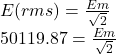Em = 70880.18 N/m

and

force on the base due to the radiation is by the radiation pressure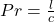………………4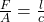so

F =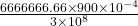F = 2 ×N